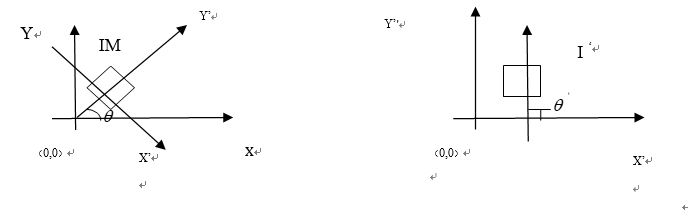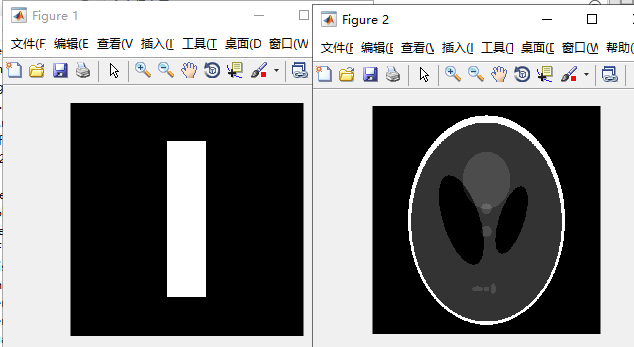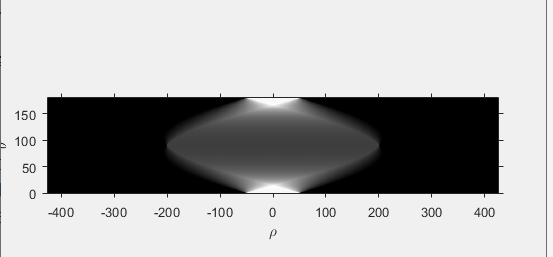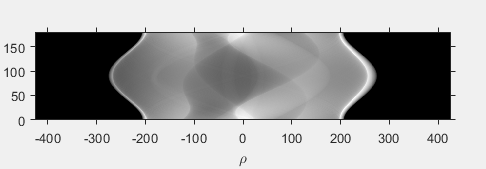2017-03-14 10:55:59 qq278672818 阅读数 3063

2016-06-29 13:15:38 lpsl1882 阅读数 11470

从一个角度，用光源照射对象物体，屏幕上会形成对象物体的影子；如果物体是半透明的，那么影子便有灰度而不是纯黑的，这说明屏幕上的像可以反映物体内部对可见光的衰减作用。我们从落于[0~π$\pi$]的一系列连续角度照射物体，形成一系列的像，这些像包含物体结构特征信息，基本上可以通过这些像还原物体的形状特征，如果物体是半透明的，那么物体内部的结构也可以还原出来。物体原始形状变换生成这些投影像，称为radon变换；从这些像还原物体形态，称为逆radon变换。人体对可见光是不透明的，但对X光是半透明的，因此CT可以发射X光照射人体，生成人体内部结构的图像信息。

xcosθ+ysinθ=ρg(ρ,θ)=f(x,y)δ(xcosθ+ysinθρ)dxdy

f(x,y)=π0g(xcosθ+ysinθ,θ)dθ

为了得到清晰的图像，我们需要进行频域滤波。首先我们知道二维傅里叶变换对为：

F(u,v)=f(x,y)ej2π(ux+vy)dxdyf(x,y)=F(u,v)ej2π(ux+vy)dudv

G(ω,θ)=g(ρ,θ)ej2πωρdρ=f(x,y)δ(xcosθ+ysinθρ)ej2πωρdxdydρ=f(x,y)[δ(xcosθ+ysinθρ)ej2πωρdρ]dxdy=f(x,y)ej2πω(xcosθ+ysinθ)dxdy=F(ωcosθ,ωsinθ)

f(x,y)=F(ωcosθ,ωsinθ)dωcosθdωsinθdωcosθdωsinθ=ωdωdθf(x,y)=F(ωcosθ,ωsinθ)ωdωdθ=2π00G(ω,θ)ej2πω(xcosθ+ysinθ)ωdωdθcos(θ+π=cosθ,sin(θ+π=sinθG(ω,θ+π)=f(x,y)ej2πω(xcosθysinθ)dxdy=f(x,y)ej2π(ω)(xcosθ+ysinθ)dxdy=G(ω,θ)f(x,y)=π00G(ω,θ)ej2πω(xcosθ+ysinθ)ωdωdθ+π00G(ω,θ)ej2π(ω)(xcosθ+ysinθ)ωdωdθlet(ωt)f(x,y)=π00G(ω,θ)ej2πω(xcosθ+ysinθ)ωdωdθ+π00G(t,θ)ej2πt(xcosθ+ysinθ)tdtdθ=π0G(ω,θ)ej2πt(xcosθ+ysinθ)|ω|dωdθ

• 我们之前进行radon投影时，看到[0~π$\pi$]和[π$\pi$~ 2π$2\pi$]的θ$\theta$角度下得到的投影是互为镜像，粗看认为信息是重复的，于是贸然舍弃一半，只计算了[0~π$\pi$]角度的投影数据，这可能隐性地造成了数据不全。
• 直接进行空间积分变换，可能造成不同角度投影之间发生混淆，导致产生模糊和光晕

• 计算每个投影的一维傅里叶变换
• 使用截断的|ω|$|\omega|$项或者类似的窗口函数进行滤波，得到新的一维傅里叶变换数据
• 计算傅里叶反变换，获得原图Matlab重建结果，注意有条纹

2018-06-03 21:44:38 corilei 阅读数 5044matlab实现

clear all
clc
close all

[inputfilename,dirname] = uigetfile('*.*');
inputfilename = [dirname, inputfilename];
im = imread(inputfilename); % For example: 'input.jpg'

grayImage = rgb2gray(im);
%%%%%

%%%%% Edge detection and edge linking....
binaryImage = edge(grayImage,'canny'); % 'Canny' edge detector
binaryImage = bwmorph(binaryImage,'thicken'); % A morphological operation for edge linking
%%%%%

%%%%% Radon transform projections along 180 degrees, from -90 to +89....
% R: Radon transform of the intensity image 'grayImage' for -90:89 degrees.
% In fact, each column of R shows the image profile along corresponding angle.
% xp: a vector containing the radial coordinates corresponding to each row of 'R'.
% Negative angles correspond to clockwise directions, while positive angles
% correspond to counterclockwise directions around the center point (up-left corner).
% R1: A 1x180 vector in which, each element is equal the maximum value of Radon transform along each angle.
% This value reflects the maximum number of pixels along each direction.
% r_max: A 1x180 vector, which includes corresponding radii of 'R1'.
theta = -90:89;
imagesc(theta,xp, R); colormap(jet);
xlabel('theta (degrees)');ylabel('x''');
colorbar
%%%%%

[R1,r_max] = max(R);
theta_max = 90;
while(theta_max > 50 || theta_max<-50)
[R2,theta_max] = max(R1); % R2: Maximum Radon transform value over all angles.
% theta_max: Corresponding angle
R1(theta_max) = 0; % Remove element 'R2' from vector 'R1', so that other maximum values can be found.
theta_max = theta_max - 91;
end

correctedImage = imrotate(im,-theta_max); % Rotation correction
correctedImage(correctedImage == 0) = 255; % Converts black resgions to white regions

subplot(1,2,1), subimage(im)
subplot(1,2,2), subimage(correctedImage)

function [bw,qingxiejiao]=radontran(bwbone,bw)%radon倾斜校正

theta=1:90;
[I0,J]=find(R>=max(max(R)));%找倾斜角
qingxiejiao=90-J;
bw=imrotate(bw,qingxiejiao,'bilinear','crop');
clc;
clear all;
close all;
[fn pn fi]=uigetfile('*.*','choose a picture');
imshow(Img);title('Original image');
I = rgb2gray(Img);
I=improve_hist(I);
bw=edge(I,'canny');
theta=1:179;
[I0,J]=find(R>=max(R(:)));%J记录了倾斜角
qingxiejiao=90-J;
I1=imrotate(Img,qingxiejiao,'bilinear','crop');
subplot(1,2,1),imshow(Img);title('Original image');
subplot(1,2,2),imshow(I1);title('correct image');

B = imrotate(A,angle)

B = imrotate(A,angle,method)

'nearest'：最邻近线性插值（Nearest-neighbor interpolation）
'bilinear'： 双线性插值（Bilinear interpolation）
'bicubic'： 双三次插值（或叫做双立方插值）（Bicubic interpolation）
B = imrotate(A,angle,method,bbox)
bbox参数用于指定输出图像属性：
'crop'： 通过对旋转后的图像B进行裁剪， 保持旋转后输出图像B的尺寸和输入图像A的尺寸一样。
'loose'： 使输出图像足够大， 以保证源图像旋转后超出图像尺寸范围的像素值没有丢失。 一般这种格式产生的图像的尺寸都要大于源图像的尺寸。

2019-06-24 23:07:04 nanhuaibeian 阅读数 384

## 一、概念

该函数的基本语法：R = radon(I,theta)，其中，I 是一个二维数组，theta 是角度值的一维数组。投影包含在 R 的列中，生成的投影数等于数组 theta 中的角度数。生成的投影长到足以在射线约束旋转时跨越观察的宽度。当射线垂直于矩形数组的主对角线时会出现这种视图。换句话说，对一个大小为 MxN 的输入数组，投影的最小长度为 [M^2 + N^2]^(1/2)
函数 radon 一个更一般的语法：[R,xp] = radon(I,theta)，其中 xp 包含沿着 x’ 轴的坐标值，xp 中的值用于标注图轴。

在 CT 算法模拟中，用来生成一幅著名图像（称为 Sheep-Logan头部幻影）的一个有用函数的语法为：P = phantom(def,n)
其中，def 是一个指定所生成头部幻影类型的字符串，n 是行数和列数（默认值为256）

def 的有效值：
‘Sheep-Logan’：计算机断层研究 人员广泛使用的测试图像。这幅图像的对比度很低。
‘Modified Shepp-Logan’：Sheep-Logan 幻影的变体，其对比度得到了改进，因此有更好的视觉效果


% 创建一个全零的数组，600 x 600
>> g1 = zeros(600,600);
% 将 g1 第100-500行，250-350 列赋值为1
>> g1(100:500,250:350) = 1;
% 生成 Shepp-Logan 头部幻影
>> g2 = phantom('Modified Shepp-Logan',600);
>> imshow(g1),figure,imshow(g2)使用半度增量的雷登变换由下面的语句得到：

% 使用 0.5 的角度增量，从 0 度到175.5度为增量
>> theta = 0:0.5:179.5;
% 对给定的二维矩形数组生成一组平行射线投影


此时，R1 的第一列是 θ = 0度，第二列是 θ = 0.5度，等等。
第一列的第一个元素对应于 ρ 的最小的负值，而最后一个元素对应于 ρ 的最大的正值。其他列类似
如果我们想要显示 R1 以便投影从左到右进行，并且第一个投影出现在图像的底部，必须转置并反转数组

% 先转置数组，然后翻转数组
>> R1 = flipud(R1');
>> R2 = flipud(R2');
% 设置显示图像的参数
>> figure,imshow(R1,[],'XData',xp1([1 end]),'YData',[179.5 0])
% 将坐标轴系统的原点由其默认的左上角移到右下角
>> axis xy
% 显示 X 轴和 Y 轴
>> axis on
% 设置 X轴为 θ轴， Y 轴为 ρ 轴
>> xlabel('\rho'),ylabel('\theta')


R1 的雷登变换图>> figure,imshow(R2,[],'XData',xp2([1 end]),'YData',[179.5 0])
>> axis xy
>> axis on
>> xlabel('\rho'),ylabel('\theta')


R2 的雷登变换图最后的这两幅图显示了结果，在这两幅图像中，每行表示了固定值 θ 的一个完整投影。

2013-12-19 11:32:47 utimes 阅读数 8282

clear all;
close all;
clc;

grayPic =rgb2gray(sourcPic);
ed = edge(grayPic,'canny');
subplot(2,2,1);
imshow(ed),title('sourcPic');

subplot(2,2,2);

subplot(2,2,3);

subplot(2,2,4);
plot(r2),title('Radon 45');
实验结果参考资料

 Radon Transform From Mathoworld Wolfrom.

=========

关于Image Engineering & Computer Vision的更多讨论与交流，敬请关注本博和新浪微博songzi_tea.

阅读数 8056

博文 来自： rain_promise

阅读数 448

博文 来自： OOFFrankDura

阅读数 260

博文 来自： lilu0719

阅读数 1357

博文 来自： u014119145

阅读数 3159

博文 来自： ljj583905183
没有更多推荐了，返回首页